## Section Exercises

1. Terry is skiing down a steep hill. Terry’s elevation, E(t), in feet after t seconds is given by $E\left(t\right)=3000-70t$. Write a complete sentence describing Terry’s starting elevation and how it is changing over time.

2. Maria is climbing a mountain. Maria’s elevation, E(t), in feet after t minutes is given by $E\left(t\right)=1200+40t$. Write a complete sentence describing Maria’s starting elevation and how it is changing over time.

3. Jessica is walking home from a friend’s house. After 2 minutes she is 1.4 miles from home. Twelve minutes after leaving, she is 0.9 miles from home. What is her rate in miles per hour?

4. Sonya is currently 10 miles from home and is walking farther away at 2 miles per hour. Write an equation for her distance from home t hours from now.

5. A boat is 100 miles away from the marina, sailing directly toward it at 10 miles per hour. Write an equation for the distance of the boat from the marina after t hours.

6. Timmy goes to the fair with $40. Each ride costs$2. How much money will he have left after riding $n$ rides?

For the following exercises, determine whether the equation of the curve can be written as a linear function.

7. $y=\frac{1}{4}x+6$

8. $y=3x - 5$

9. $y=3{x}^{2}-2$

10. $3x+5y=15$

11. $3{x}^{2}+5y=15$

12. $3x+5{y}^{2}=15$

13. $-2{x}^{2}+3{y}^{2}=6$

14. $-\frac{x - 3}{5}=2y$

For the following exercises, determine whether each function is increasing or decreasing.

15. $f\left(x\right)=4x+3$

16. $g\left(x\right)=5x+6$

17. $a\left(x\right)=5 - 2x$

18. $b\left(x\right)=8 - 3x$

19. $h\left(x\right)=-2x+4$

20. $k\left(x\right)=-4x+1$

21. $j\left(x\right)=\frac{1}{2}x - 3$

22. $p\left(x\right)=\frac{1}{4}x - 5$

23. $n\left(x\right)=-\frac{1}{3}x - 2$

24. $m\left(x\right)=-\frac{3}{8}x+3$

For the following exercises, find the slope of the line that passes through the two given points.

25. $\left(2,\text{ }4\right)$ and $\left(4,\text{ 10}\right)$

26. $\left(1,\text{ 5}\right)$ and $\left(4,\text{ 11}\right)$

27. $\left(-1,\text{4}\right)$ and $\left(5,\text{2}\right)$

28. $\left(8,-2\right)$ and $\left(4,6\right)$

29. $\left(6,\text{ }11\right)$ and $\left(-4, 3\right)$

For the following exercises, given each set of information, find a linear equation satisfying the conditions, if possible.

30. $f\left(-5\right)=-4$, and $f\left(5\right)=2$

31. $f\left(-1\right)=4$ and $f\left(5\right)=1$

32. $\left(2,4\right)$ and $\left(4,10\right)$

33. Passes through $\left(1,5\right)$ and $\left(4,11\right)$

34. Passes through $\left(-1,\text{ 4}\right)$ and $\left(5,\text{ 2}\right)$

35. Passes through $\left(-2,\text{ 8}\right)$ and $\left(4,\text{ 6}\right)$

36. x intercept at $\left(-2,\text{ 0}\right)$ and y intercept at $\left(0,-3\right)$

37. x intercept at $\left(-5,\text{ 0}\right)$ and y intercept at $\left(0,\text{ 4}\right)$

For the following exercises, find the slope of the lines graphed.

38.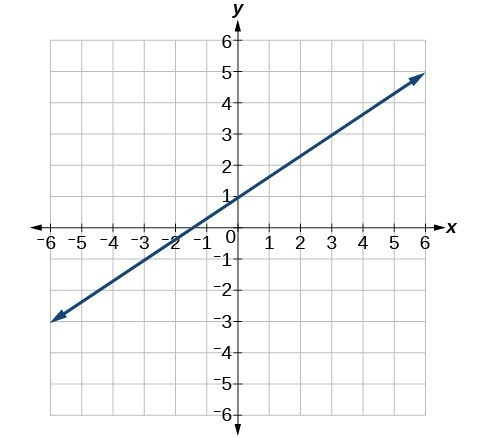39.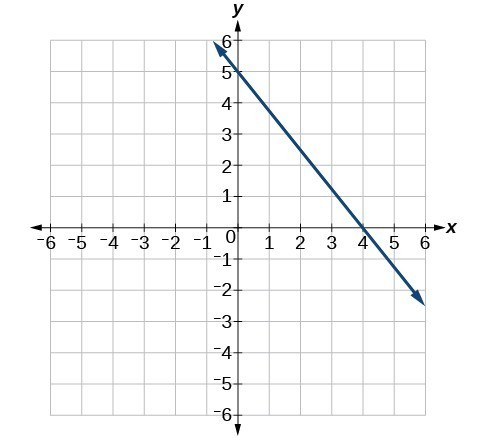40.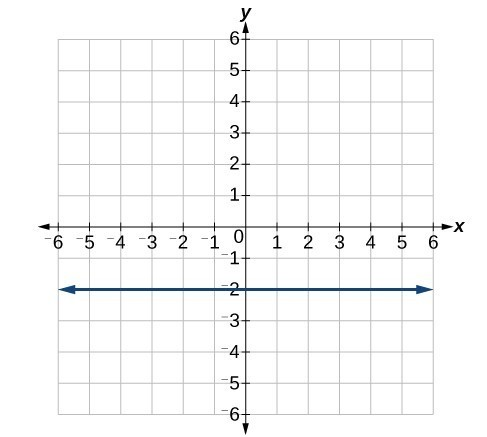For the following exercises, write an equation for the lines graphed.

41.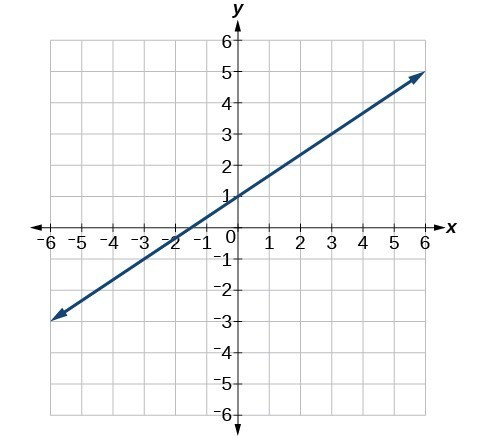42.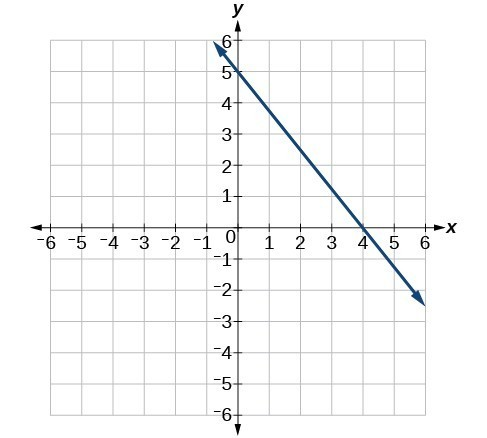43.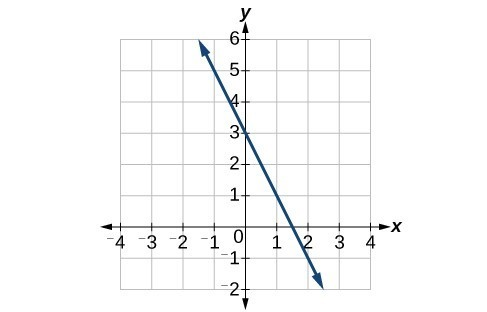44.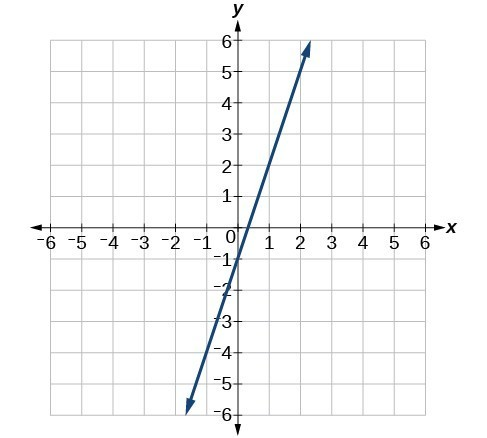45.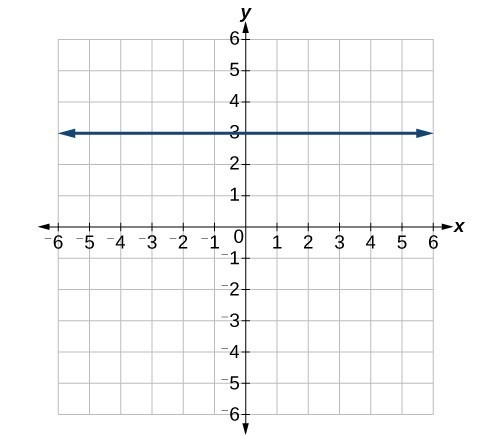46.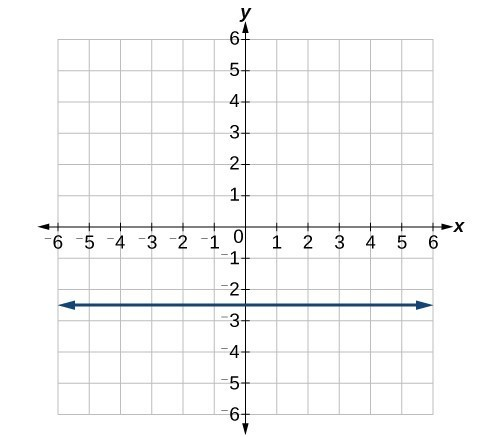For the following exercises, which of the tables could represent a linear function? For each that could be linear, find a linear equation that models the data.

47.

 x 0 5 10 15 g(x) 5 –10 –25 –40

48.

 x 0 5 10 15 h(x) 5 30 105 230

49.

 x 0 5 10 15 f(x) –5 20 45 70

50.

 x 5 10 20 25 k(x) 28 13 58 73

51.

 x 0 2 4 6 g(x) 6 –19 –44 –69

52.

 x 2 4 6 8 f(x) –4 16 36 56

53.

 x 2 4 6 8 f(x) –4 16 36 56

54.

 x 0 2 6 8 k(x) 6 31 106 231

55. If is a linear function, $f\left(0.1\right)=11.5, \text{ and} f\left(0.4\right)=-5.9$, find an equation for the function.

56. Graph the function f on a domain of $\left[-10, 10\right]: f\left(x\right)=0.02x - 0.01$. Enter the function in a graphing utility. For the viewing window, set the minimum value of to be –10 and the maximum value of x to be 10.

57. Graph the function f on a domain of $\left[-10, 10\right]:f\left(x\right)=2,500x+4,000$

58. The table below shows the input, w, and output, k, for a linear function k. a. Fill in the missing values of the table. b. Write the linear function k, round to 3 decimal places.

 w –10 5.5 67.5 b k 30 –26 a –44

59. shows the input, p, and output, q, for a linear function q. a. Fill in the missing values of the table. b. Write the linear function k.

 p 0.5 0.8 12 b q 400 700 a 1,000,000

60. Graph the linear function f on a domain of [–10, 10] for the function whose slope is $\frac{1}{8}$ and y-intercept is $\frac{31}{16}$. Label the points for the input values of –10 and 10.

61. Graph the linear function f on a domain of [–0.1, 0.1] for the function whose slope is 75 and y-intercept is –22.5. Label the points for the input values of –0.1 and 0.1.

62. Graph the linear function f where $f\left(x\right)=ax+b$ on the same set of axes on a domain of [–4, 4] for the following values of a and b.

$a=2;\text{ }b=3$
$a=2;\text{ }b=4$
$a=2;\text{ }b=-4$
$a=2;\text{ }b=-5$

63. Find the value of x if a linear function goes through the following points and has the following slope: $\left(x,2\right),\left(-4,6\right),m=3$

64. Find the value of y if a linear function goes through the following points and has the following slope: $\left(10,y\right),\left(25,100\right),m=-5$

65. Find the equation of the line that passes through the following points: $\left(a,\text{ }b\right)$ and $\left(a,\text{ }b+1\right)$

66. Find the equation of the line that passes through the following points: $\left(2a,\text{ }b\right)$ and $\left(a,\text{ }b+1\right)$

67. Find the equation of the line that passes through the following points: $\left(a,\text{ }0\right)$ and $\left(c,\text{ }d\right)$

68. At noon, a barista notices that she has $20 in her tip jar. If she makes an average of$0.50 from each customer, how much will she have in her tip jar if she serves n more customers during her shift?

69. A gym membership with two personal training sessions costs $125, while gym membership with five personal training sessions costs$260. What is cost per session?

70. A clothing business finds there is a linear relationship between the number of shirts, n, it can sell and the price, p, it can charge per shirt. In particular, historical data shows that 1,000 shirts can be sold at a price of $30, while 3,000 shirts can be sold at a price of$22. Find a linear equation in the form $p\left(n\right)=mn+b$ that gives the price p they can charge for n shirts.

71. A phone company charges for service according to the formula: $C\left(n\right)=24+0.1n$, where n is the number of minutes talked, and $C\left(n\right)$ is the monthly charge, in dollars. Find and interpret the rate of change and initial value.

72. A farmer finds there is a linear relationship between the number of bean stalks, n, she plants and the yield, y, each plant produces. When she plants 30 stalks, each plant yields 30 oz of beans. When she plants 34 stalks, each plant produces 28 oz of beans. Find a linear relationships in the form $y=\mathrm{mn}+b$ that gives the yield when n stalks are planted.

73. A city’s population in the year 1960 was 287,500. In 1989 the population was 275,900. Compute the rate of growth of the population and make a statement about the population rate of change in people per year.

74. A town’s population has been growing linearly. In 2003, the population was 45,000, and the population has been growing by 1,700 people each year. Write an equation, $P\left(t\right)$, for the population t years after 2003.

75. Suppose that average annual income (in dollars) for the years 1990 through 1999 is given by the linear function: $I\left(x\right)=1054x+23,286$, where x is the number of years after 1990. Which of the following interprets the slope in the context of the problem?

a. As of 1990, average annual income was $23,286. b. In the ten-year period from 1990–1999, average annual income increased by a total of$1,054.
c. Each year in the decade of the 1990s, average annual income increased by $1,054. d. Average annual income rose to a level of$23,286 by the end of 1999.

76. When temperature is 0 degrees Celsius, the Fahrenheit temperature is 32. When the Celsius temperature is 100, the corresponding Fahrenheit temperature is 212. Express the Fahrenheit temperature as a linear function of C, the Celsius temperature, $F\left(C\right)$.

a. Find the rate of change of Fahrenheit temperature for each unit change temperature of Celsius.
b. Find and interpret $F\left(28\right)$.
c. Find and interpret $F\left(-40\right)$.